﻿ 利用CEEMDAN进行GNSS-MR雪深反演文章快速检索 高级检索
 大地测量与地球动力学2022, Vol. 42Issue (1): 25-28  DOI: 10.14075/j.jgg.2022.01.006### 引用本文XUE Zhangfang, LIU Lilong, WU Haojian, et al. GNSS-MR Snow Depth Inversion Based on CEEMDAN[J]. Journal of Geodesy and Geodynamics, 2022, 42(1): 25-28.### Foundation support

National Natural Science Foundation of China, No. 42064002, 41664002; Guangxi Natural Science Foundation, No. 2018GXNSFAA294045.

### Corresponding author

LIU Lilong, PhD, professor, majors in GNSS technology and application, E-mail: hn_liulilong@163.com.

### 第一作者简介

XUE Zhangfang, postgraduate, majors in GNSS-R remote sensing theory and method, E-mail: xzf13080153313@163.com.

### 文章历史

1. 桂林理工大学测绘地理信息学院，桂林市雁山街319号，541006;
2. 广西空间信息与测绘重点实验室，桂林市雁山街319号，541006

1 GNSS-MR反演雪深原理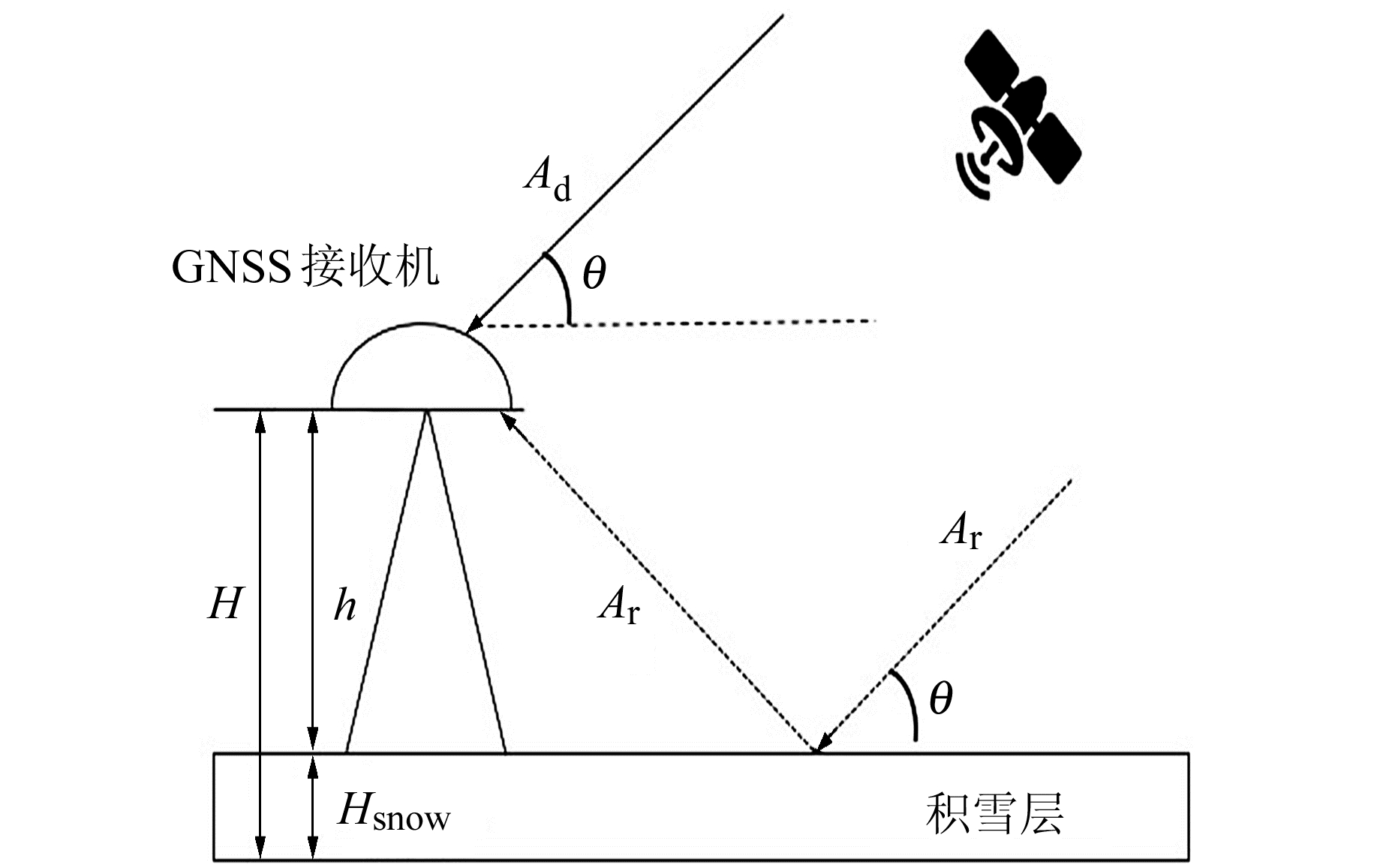图 1 GNSS-MR雪深探测示意图 Fig. 1 Diagram of GNSS-MR for snow depth

 ${A_{\rm{d}}} \gg {A_{\rm{r}}}$ (1)

Ac为合成信号的振幅, φ为直射信号与经地表积雪面反射的信号的夹角，其关系为

 ${\rm{SN}}{{\rm{R}}^2} = A_{\rm{c}}^2 = A_{\rm{d}}^2 + A_{\rm{r}}^2 + 2{A_{\rm{d}}}{A_{\rm{r}}}{\rm{cos}}\varphi$ (2)

 ${A_{\rm{r}}} = {A_{\rm{d}}}{\rm{cos}}\left( {\frac{{4{\rm{ \mathsf{ π} }}h}}{\lambda }{\rm{sin}}\theta + \varphi } \right)$ (3)

2 CEEMDAN

CEEMDAN是对经验模态分解(empirical mode decomposition, EMD)改进后得到的一种变换形式, 其每个本征模态函数(intrinsic mode function, IMF)对应一个频率，具有各自的物理意义。因此，可通过CEEMDAN将原始SNR信号分解成各阶IMF并重构，从而获取高质量的残差序列。

1) 与EEMD算法相同，在x(t) 中加入白噪声εkωi(t)，对其进行EMD分解得到多个IMF分量并求集合平均值。第1阶最终分量IMF1(t)为：

 ${\rm{IM}}{{\rm{F}}_1}\left( t \right) = \frac{1}{I}\sum\limits_{i = 1}^I {{E_1}\left[ {x\left( t \right) + {\varepsilon _0}{\omega _i}\left( t \right)} \right]}$ (4)

2) 当k=1时，计算1阶残差r1(t):

 ${r_1}\left( t \right) = x\left( t \right) - {\rm{IM}}{{\rm{F}}_1}\left( t \right)$ (5)

3) 对1阶残差r1(t) 添加经EMD分解后的噪声分量，得到一个新信号，即r1(t)+ε1E1[ωi(t)]，再对其分解，得到的集合平均值为该阶的IMF最终分量：

 $\begin{array}{l} \;\;\;\;\;\;\;\;\;\;{\rm{IM}}{{\rm{F}}_2}\left( t \right) = \\ \frac{{\rm{1}}}{I}\sum\limits_{i = 1}^I {} {E_1}\left[ {{r_1}\left( t \right) + {\varepsilon _1}{E_1}\left[ {{\omega _i}\left( t \right)} \right]} \right] \end{array}$ (6)

4) 当k=2，…，K时, 计算第k阶残差rk(t)：

 ${r_k}\left( t \right) = {r_{k - 1}}\left( t \right){\rm{ - IM}}{{\rm{F}}_k}\left( t \right)$ (7)

5) 对k阶残差rk(t) 添加噪声分量εkEk[ωi(t)]，获得一个新信号，计算集合平均值，得第k+1阶的最终IMF为：

 $\begin{array}{l} \;\;\;\;\;\;\;\;\;\;\;\;{\rm{IM}}{{\rm{F}}_{k + 1}}\left( t \right) = \\ \frac{1}{I}\sum\limits_{i = 1}^I {} {E_1}\left[ {{r_k}\left( t \right) + {\varepsilon _k}{E_k}\left[ {{\omega _i}\left( t \right)} \right]} \right] \end{array}$ (8)

6) 重复第4步，直到获得的残差不可再被分解。残差最后满足：

 $R\left( t \right) = x\left( t \right) - \sum\limits_{k = 1}^K {} {\rm{IM}}{{\rm{F}}_k}\left( t \right)$ (9)

x(t) 最终被分解为K个IMF分量和1个残余分量：

 $x\left( t \right) = \sum\limits_{k = 1}^K {} {\rm{IM}}{{\rm{F}}_k}\left( t \right) + R\left( t \right)$ (10)
3 实验分析

 $\left\{ \begin{array}{l} {\rm{ME}} = \frac{{\sum\limits_{i = 1}^n {} \left( {{X_1}\left( i \right) - {X_2}\left( i \right)} \right)}}{n}\\ {\rm{RMSE}} = \sqrt {\frac{{\sum\limits_{i = 1}^n {} {\rm{ }}{{({X_1}\left( i \right) - {X_2}\left( i \right))}^2}}}{n}} \\ R = \\ \frac{{\sum\limits_{i = 1}^n {} {\rm{ }}({X_1}\left( i \right) - \bar X{_1}\left( i \right))({X_2}\left( i \right) - {{\bar X}_2}\left( i \right))}}{{\sum\limits_{i = 1}^n {} {{({X_1}\left( i \right) - \bar X{_1}\left( i \right))}^2}\sum\limits_{i = 1}^n {} {{({X_2}\left( i \right) - \bar X{_2}\left( i \right))}^2}}} \end{array} \right.$ (11)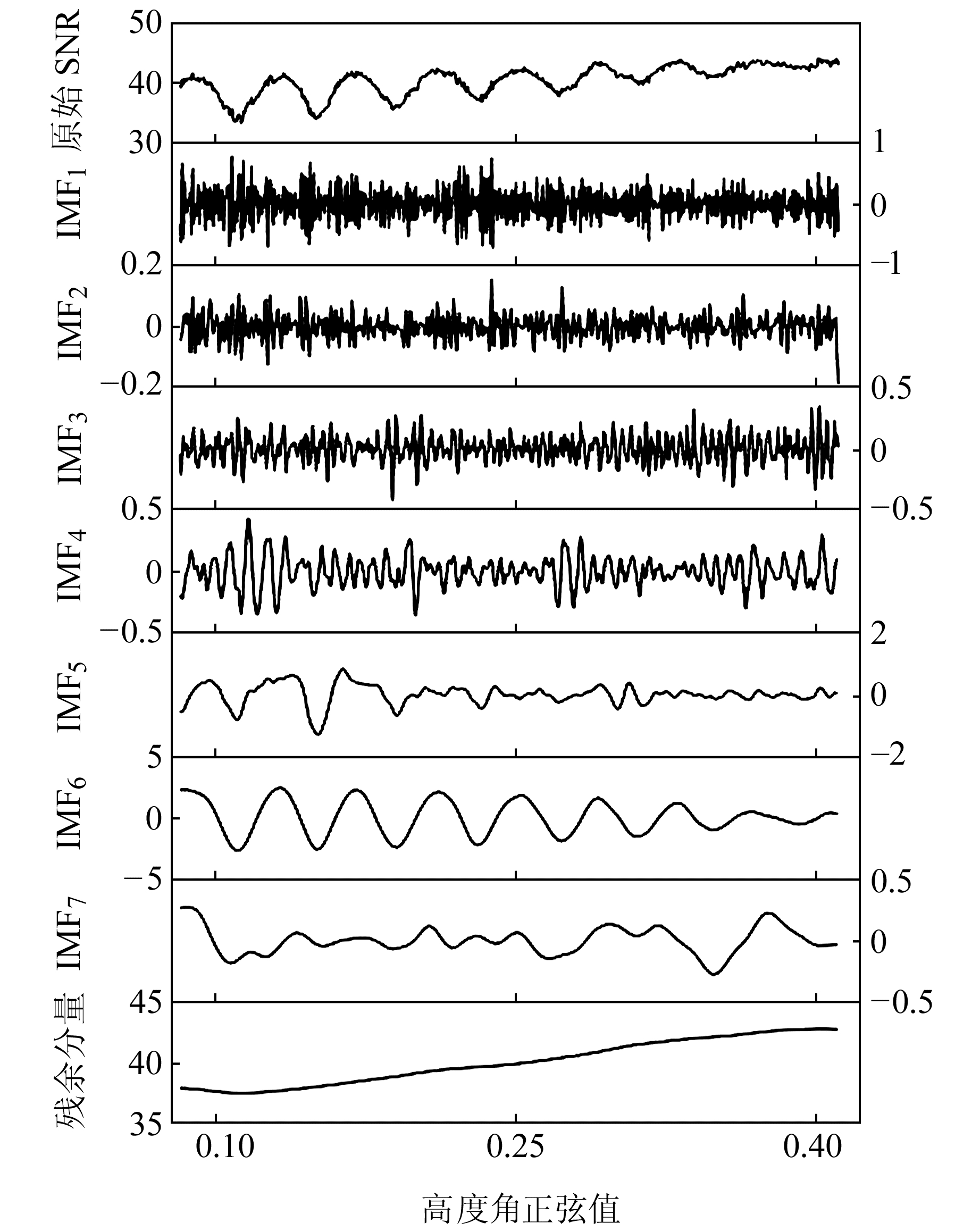图 2 CEEMDAN分解SNR序列 Fig. 2 Decomposition of SNR sequence by CEEMDAN表 1 不同IMF分量与原始信号的相关系数 Tab. 1 Correlation coefficients between different IMF components and the original signal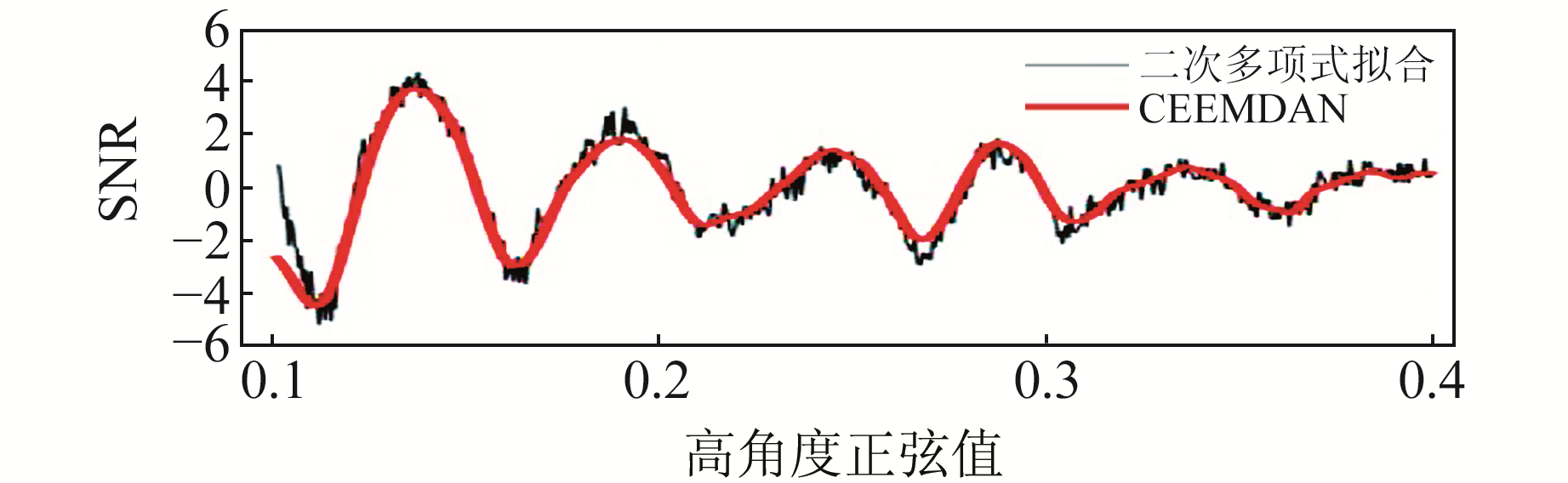图 3 2种方法获得的残差序列 Fig. 3 Residual sequences obtained by two methods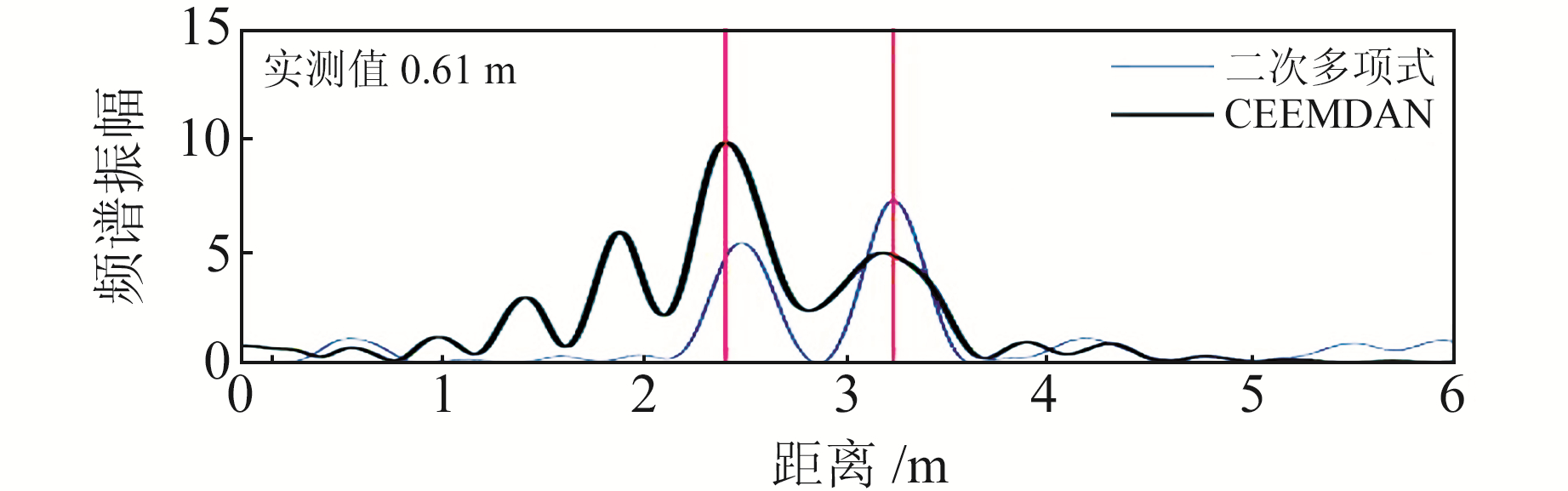图 4 SNR残差序列Lomb-Scargle谱分析图 Fig. 4 Lomb-Scargle spectrum analysis diagram of SNR residual sequence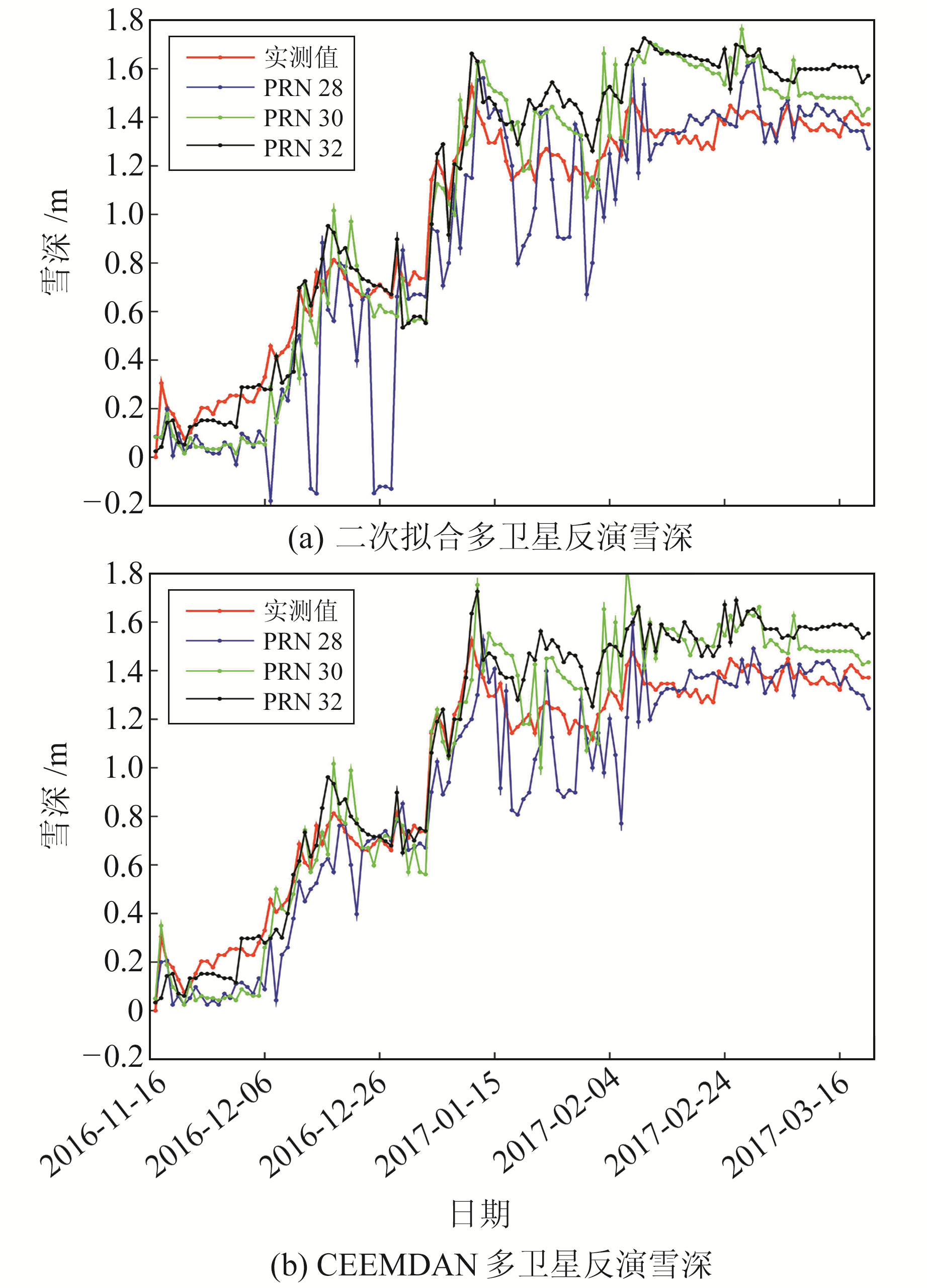图 5 2种方法多卫星反演雪深与实测雪深的对比 Fig. 5 Comparison between actual snow depth and multi-satellite inversion of snow depth based on two methods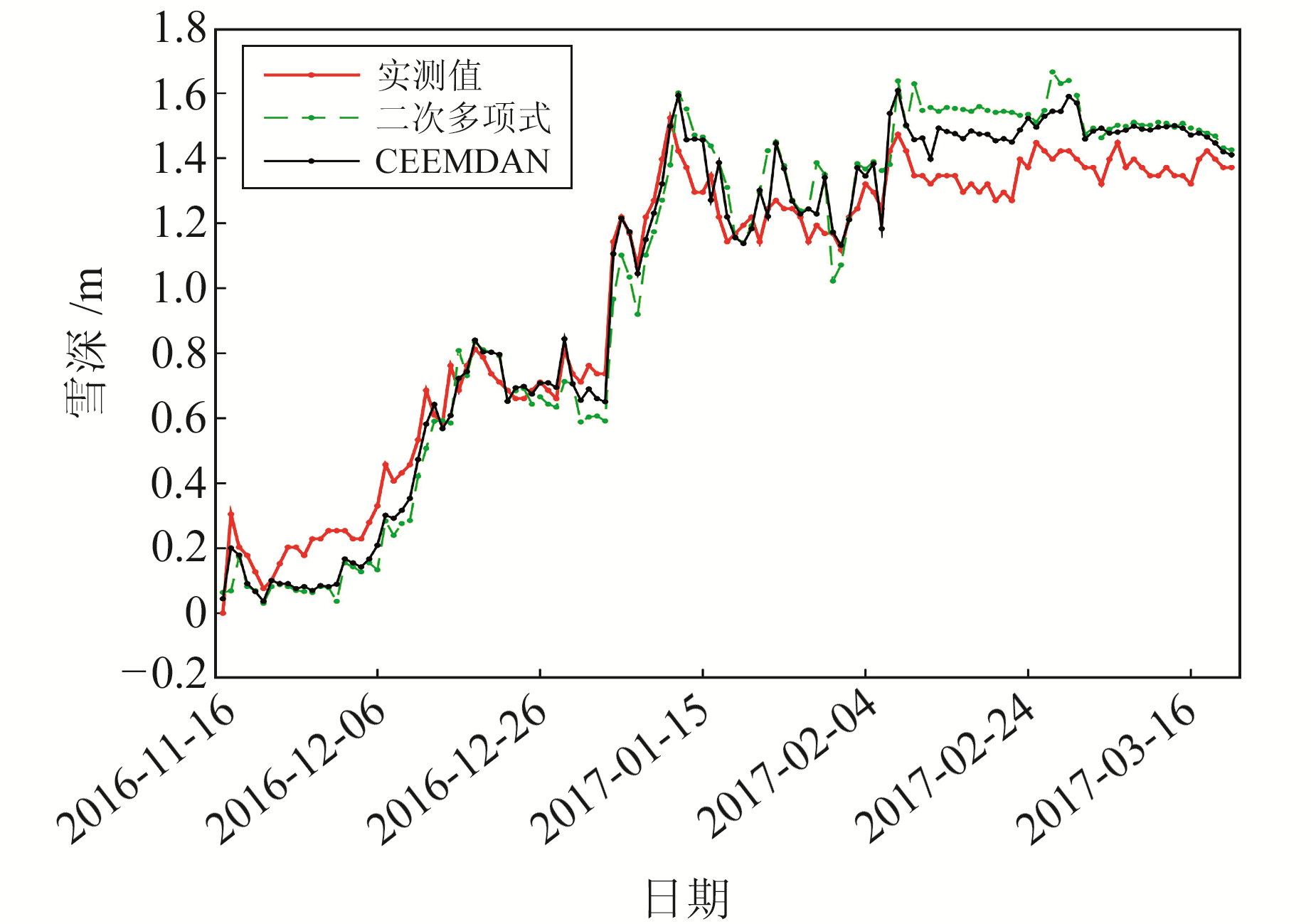图 6 2种方法多卫星融合反演雪深与实测雪深 Fig. 6 Comparison between actual snow depth and multi-satellite fusion inversion results of snow depth based on two methods表 2 2种方法反演雪深与实际雪深的平均误差与均方根误差 Tab. 2 ME and RMSE between the retrieved snow depth and the actual snow depth based on two methods
4 结语

1) CEEMDAN分解得到的残余分量可准确体现原始SNR信号的趋势，由实验数据分析可知，该方法可有效改善二次多项式拟合方法出现的异常跳变现象。

2) 本文方法可精确地提取SNR反射信号与直射信号的干涉信号，反演结果与实测数据的相关系数大于0.96，RMSE比使用传统二次多项式法降低30.7%，ME优于3 cm，验证了该方法用于GNSS-MR雪深反演的优越性。

  张双成, 戴凯阳, 南阳, 等. GNSS-MR技术用于雪深探测的初步研究[J]. 武汉大学学报: 信息科学版, 2018, 43(2): 234-240 (Zhang Shuangcheng, Dai Kaiyang, Nan Yang, et al. Preliminary Research on GNSS-MR for Snow Depth[J]. Geomatics and Information Science of Wuhan University, 2018, 43(2): 234-240) (0)  Ozeki M, Heki K. GPS Snow Depth Meter with Geometry-Free Linear Combinations of Carrier Phases[J]. Journal of Geodesy, 2012, 86(3): 209-219 DOI:10.1007/s00190-011-0511-x (0)  Torres M E, Colominas M A, Schlotthauer G, et al. A Complete Ensemble Empirical Mode Decomposition with Adaptive Noise[C]. International Conference on Acoustics, Speech and Signal Processing, Prague, 2011 (0)  Bilich A, Larson K M. Mapping the GPS Multipath Environment Using the Signal-to-Noise Ratio(SNR)[J]. Radio Science, 2007, 42(6) (0)  Huang N E, Shen Z, Long S R, et al. The Empirical Mode Decomposition and the Hilbert Spectrum for Nonlinear and Non-Stationary Time Series Analysis[J]. Proceedings of the Royal Society of London Series A: Mathematical, Physical and Engineering Sciences, 1998, 454(1 971): 903-995 (0)  周先春, 嵇亚婷. 基于EEMD算法在信号去噪中的应用[J]. 电子设计工程, 2014, 22(8): 12-14 (Zhou Xianchun, Ji Yating. Methods Based on EEMD in Signal De-Noising[J]. Electronic Design Engineering, 2014, 22(8): 12-14 DOI:10.3969/j.issn.1674-6236.2014.08.004) (0)  林丽, 余轮. 基于相关系数的EMD改进算法[J]. 计算机与数字工程, 2008, 36(12): 28-29 (Lin Li, Yu Lun. Improvement on Empirical Mocomposition Based on Correlation Coefficient[J]. Computer and Digital Engineering, 2008, 36(12): 28-29) (0)
GNSS-MR Snow Depth Inversion Based on CEEMDAN
XUE Zhangfang1,2LIU Lilong1,2WU Haojian1     ZHANG Zhi1     LIU Ruiguo1
1. College of Geomatics and Geoinformation, Guilin University of Technology, 319 Yanshan Street, Guilin 541006, China;
2. Guangxi Key Laboratory of Spatial Information and Geomatics, 319 Yanshan Street, Guilin 541006, China
Abstract: This paper introduces CEEMDAN to replace the commonly used quadratic polynomial method to decompose the original signal-to-noise ratio signal, directly extract the corresponding intrinsic mode function, and then use GNSS-MR technology to retrieve snow depth. Experiments with Colorado NWOT station GPS data show that, compared with the traditional method, the root mean square error of this method is decreased by 30.7% and the correlation coefficient with actual snow depth is 0.965, which verifies the effectiveness of the method in this article.
Key words: GNSS-MR; CEEMDAN; multipath effect; snow depth